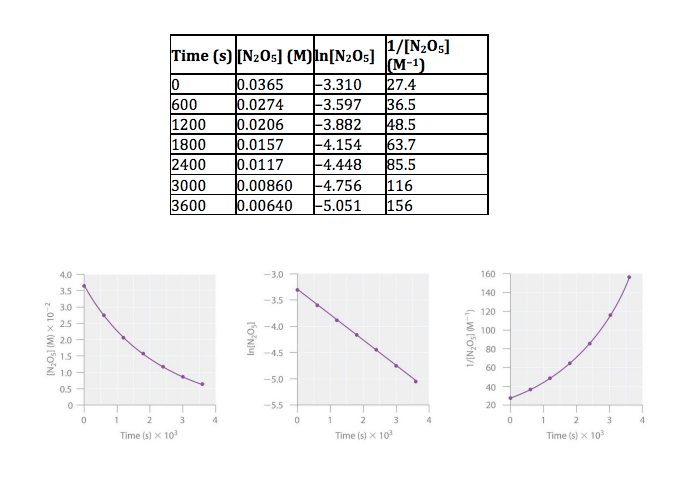Chemistry Practice Problems Identifying Reaction Order Practice Problems Solution: Dinitrogen pentoxide (N2O5) decomposes to NO2 and ...

🤓 Based on our data, we think this question is relevant for Professor Langowski's class at RUTGERS.

# Solution: Dinitrogen pentoxide (N2O5) decomposes to NO2 and O2 at relatively low temperatures in the following reaction: 2N2O5(aq) → 4NO2(aq) + O2(g) This reaction is carried out in a CCl 4 solution at 45°C. The data are described in the table as well as the plots of the concentration versus t, ln concentration versus t, and 1/concentration versus t and results are below. Determine the rate law and calculate the rate constant. a. Zero order/ 5.3 x10-5 s -1     b. Second order/ 4.8 x 10−4 s −1 M-1      c. First order/5.3 x 10-5 s -1 M-1 d. Second order/ 2.9 x 10−4 s −1 M-1               e. First order/4.8 x 10−4 s −1

###### Problem

Dinitrogen pentoxide (N2O5) decomposes to NO2 and O2 at relatively low temperatures in the following reaction:

2N2O5(aq) → 4NO2(aq) + O2(g)

This reaction is carried out in a CCl 4 solution at 45°C. The data are described in the table as well as the plots of the concentration versus t, ln concentration versus t, and 1/concentration versus t and results are below. Determine the rate law and calculate the rate constant.

a. Zero order/ 5.3 x10-5 s -1     b. Second order/ 4.8 x 10−4 s −1 M-1      c. First order/5.3 x 10-5 s -1 M-1

d. Second order/ 2.9 x 10−4 s −1 M-1               e. First order/4.8 x 10−4 s −1Identifying Reaction Order

Identifying Reaction Order

#### Q. Fluorine-18 undergoes positron emission with a half life of 1.10x 10  2 minutes. If a patient is given a 248 mg dose for a PET scan, how long will it ...

Solved • Wed Mar 16 2016 18:11:18 GMT-0400 (EDT)

Identifying Reaction Order

#### Q. Fill in plots for the following reaction orders. Be sure to label as much as possible (axes, slopes, intercepts).

Solved • Wed Feb 24 2016 11:12:55 GMT-0500 (EST)

Identifying Reaction Order

#### Q. A reaction has a rate constant of 0.0185 s-1 and the only reactant has an initial concentration of 0.135 M. What is the concentration in molarity of t...

Solved • Sun Feb 21 2016 11:17:49 GMT-0500 (EST)

Identifying Reaction Order

#### Q. For what order reaction does the half-life get longer as the initial concentration increases? A. zeroth order B. first order C. second order D. no...

Solved • Sun Feb 21 2016 11:04:58 GMT-0500 (EST)